# Introducing 'displease' - a package for non-linear numeric inteprolationThe `displease` package provides non-linear interpolation between numeric values.

It is a replacement for `seq()` for when you want a sequence between two values which is not equally spaced.

Easing can look much better than strict linear interpolation when applied to objects moving spatially.

`displease` = displ + ease. The results are often used for animating visual displacement, and the non-linear interpolation is performed by applying an easing function.

## Installation

You can install from GitHub with:

``````# install.package('remotes')

## What’s in the box?

• `seq_ease(x1, x2, n, type)` - create a sequence of length `n` between `x1` and `x2`. Apply the easing function given by `type`.
• type is one of: `sine-in`, `sine-out`, `sine-in-out`, `quad-in`, `quad-out`, `quad-in-out`, `cubic-in`, `cubic-out`, `cubic-in-out`, `quart-in`, `quart-out`, `quart-in-out`, `quint-in`, `quint-out`, `quint-in-out`, `exp-in`, `exp-out`, `exp-in-out`, `circle-in`, `circle-out`, `circle-in-out`, `back-in`, `back-out`, `back-in-out`, `elastic-in`, `elastic-out`, `elastic-in-out`

The following graph shows the `seq_ease()` output for `n = 100` for all the different easing functions with given extens of `x1 = 0` and `x2 = 1`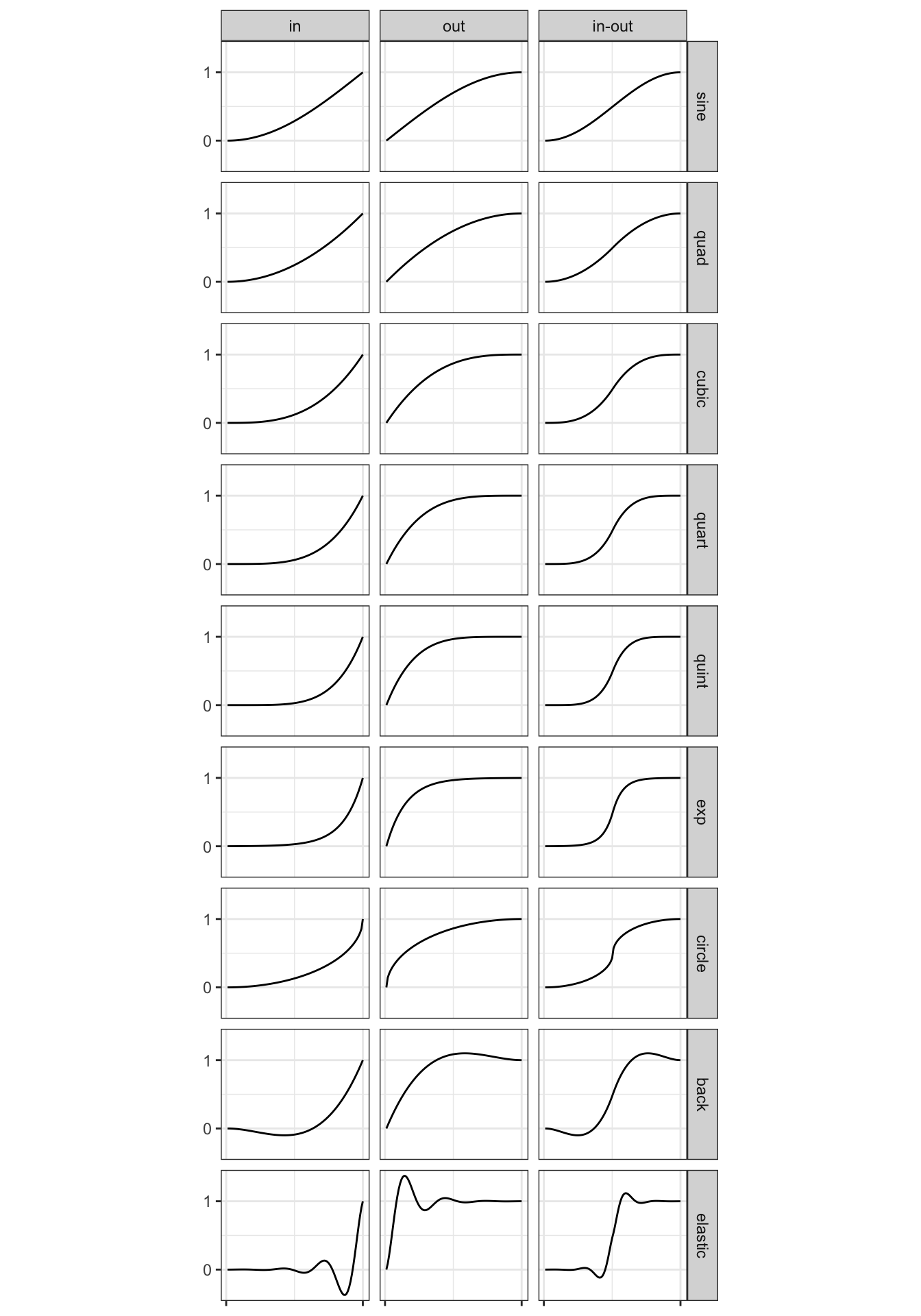## Example

``````library(displease)

# normal linear interpolation using seq()
(y_linear       <- seq(1, 10, length.out = 10))
  1  2  3  4  5  6  7  8  9 10

(y_cubic_in     <- seq_ease(1, 10, n=10, type = 'cubic-in'))
  1.000000  1.012346  1.098765  1.333333  1.790123  2.543210  3.666667
  5.234568  7.320988 10.000000
(y_cubic_in_out <- seq_ease(1, 10, n=10, type = 'cubic-in-out'))
  1.000000  1.049383  1.395062  2.333333  4.160494  6.839506  8.666667
  9.604938  9.950617 10.000000

plot(1:10, y_linear      , type = 'b')``````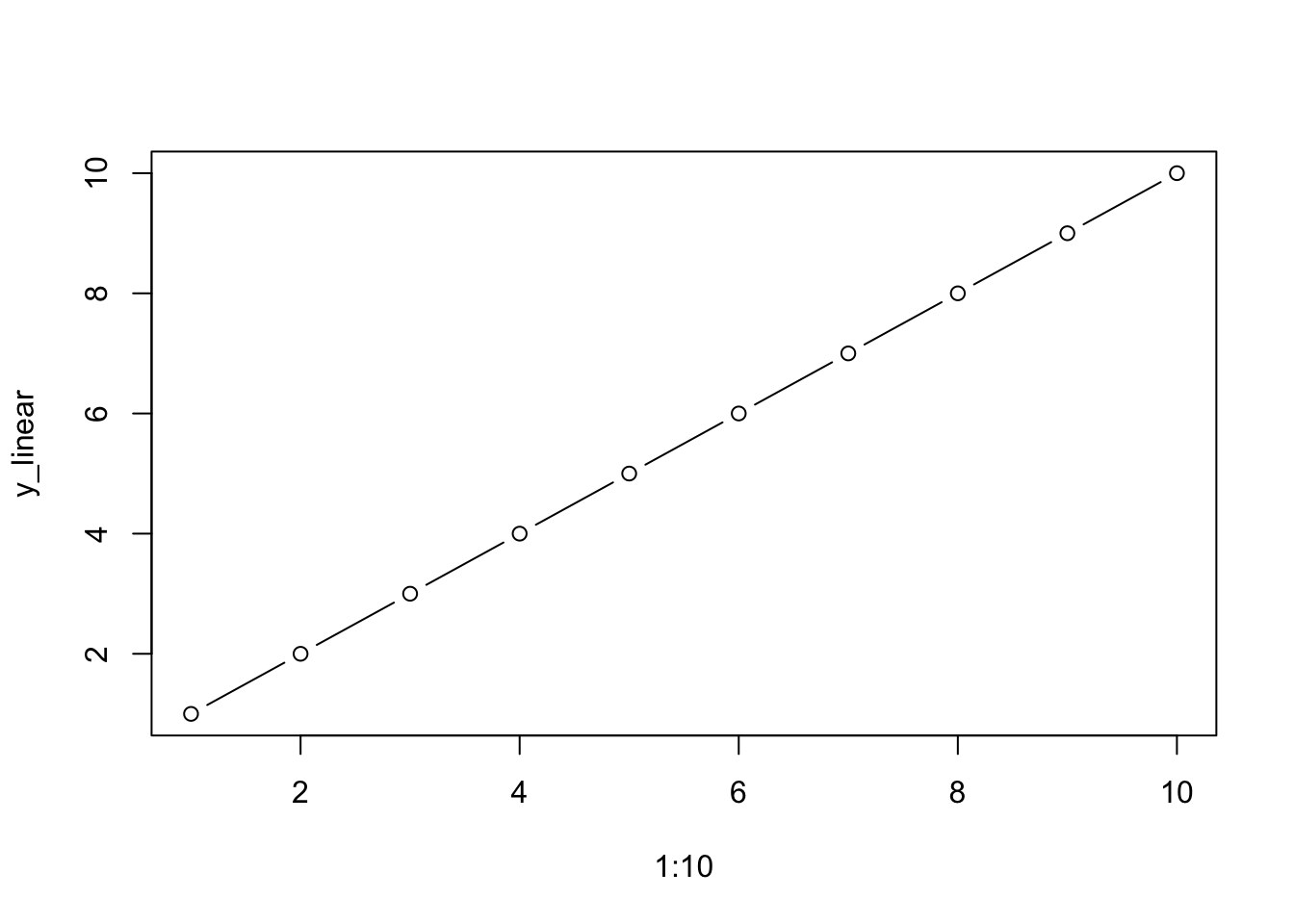``plot(1:10, y_cubic_in    , type = 'b')``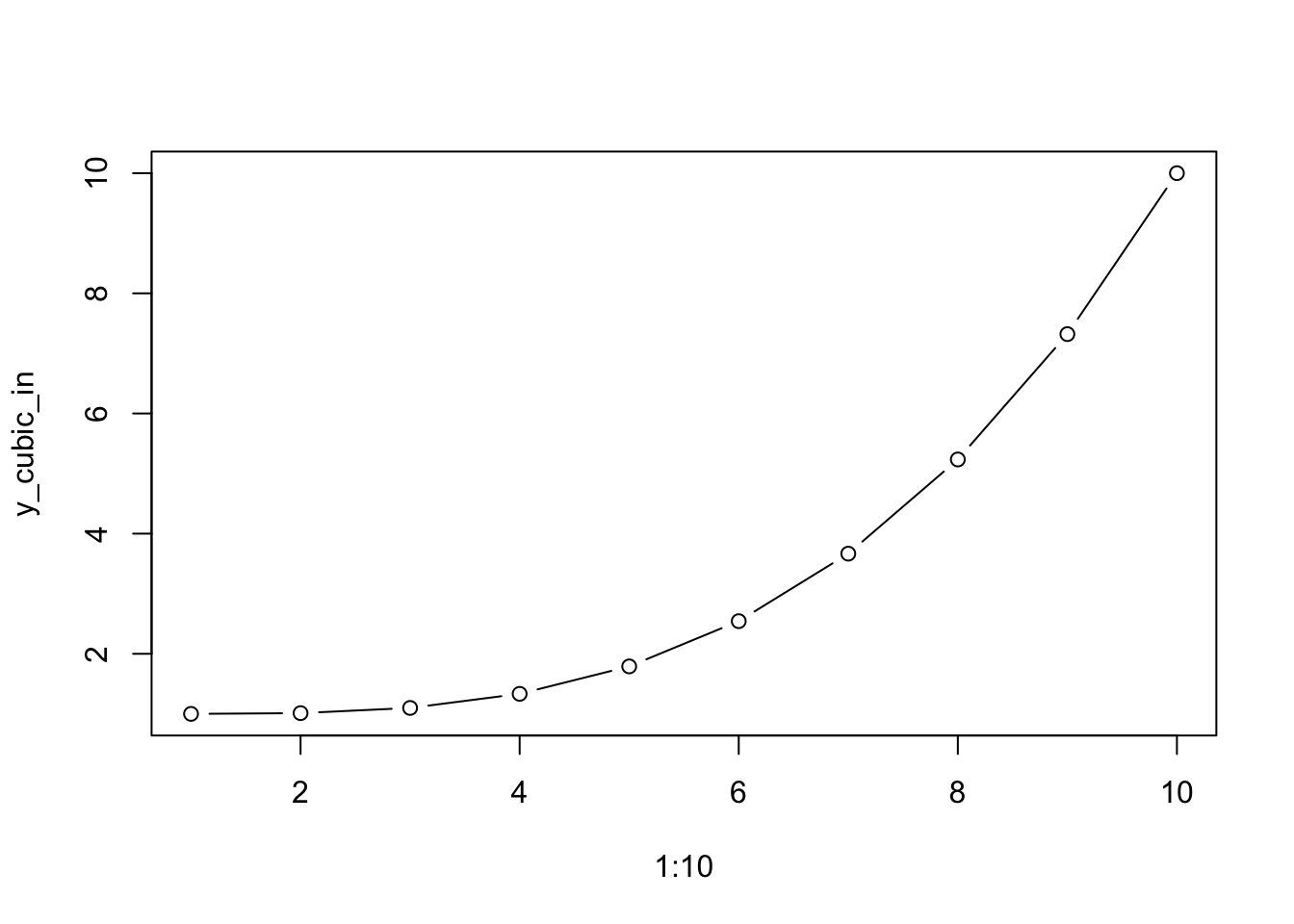``plot(1:10, y_cubic_in_out, type = 'b')``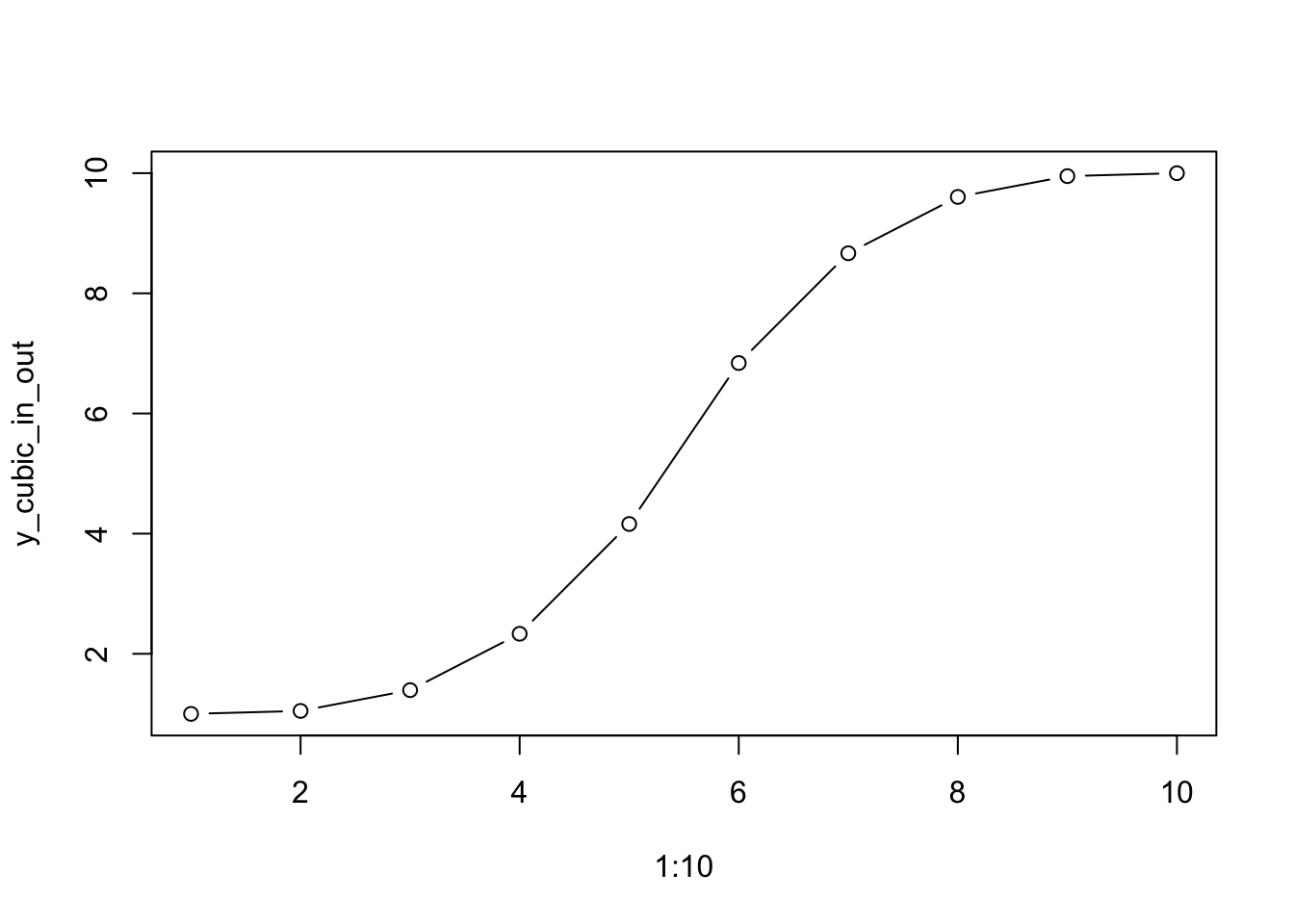## Animated example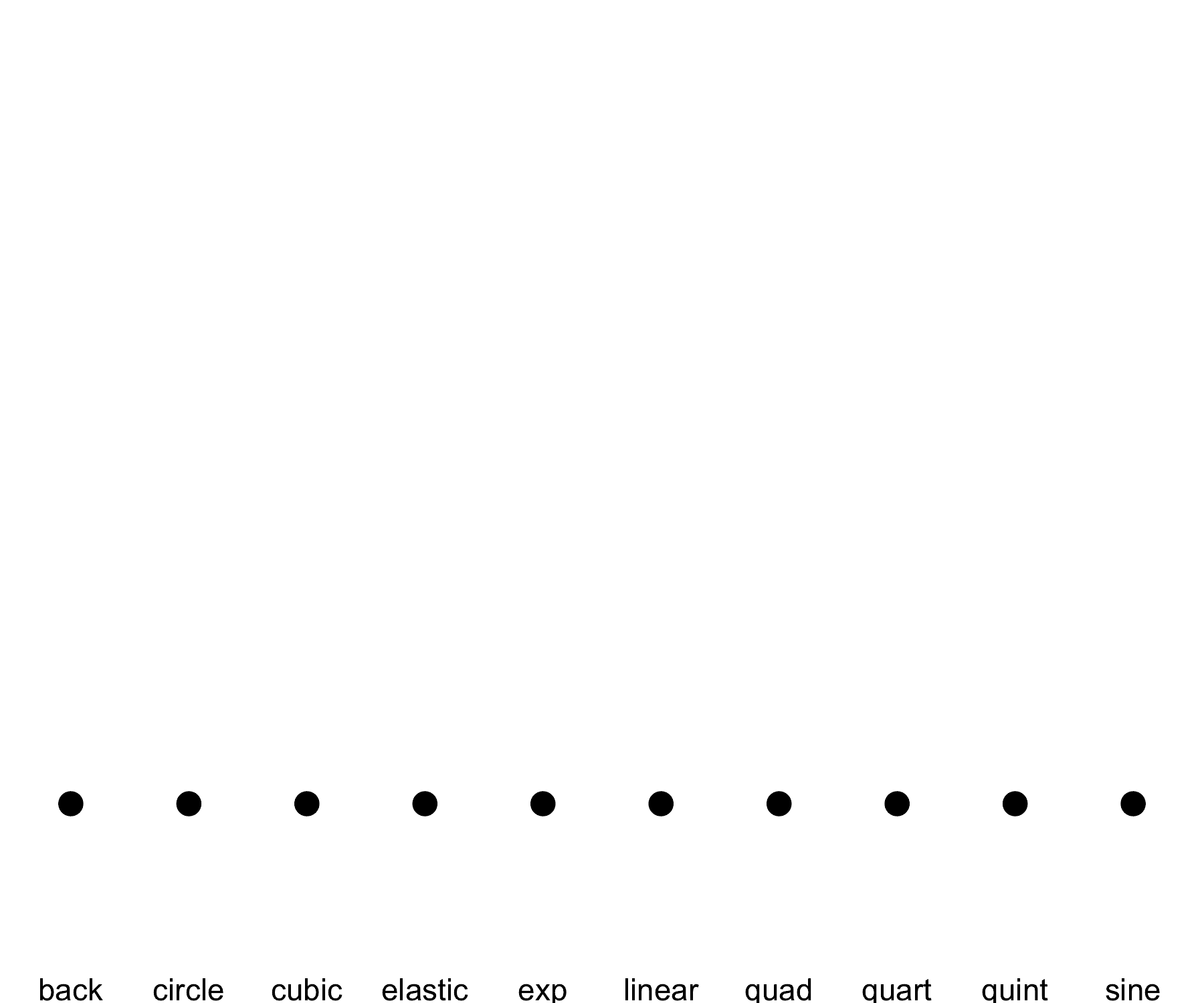## Acknowledgements

• R Core for developing and maintaining such a wonderful language.
• CRAN maintainers, for patiently shepherding packages onto CRAN and maintaining the repository